﻿ 非均匀准脆性岩土类材料的动态力学本构关系的繁衍与演化

# 非均匀准脆性岩土类材料的动态力学本构关系的繁衍与演化Propagation and Evolution of Dynamic Mechanical Constitutive Relation of Heterogeneous Quasi-Brittle Materials

Abstract: Constitutive theory describes the response of material or structure under external load at macro and micro scales. It is a research direction that cannot be ignored in mechanical research and a long-standing research topic in material mechanics. This paper focuses on non-uniform medium such as rock or rock mass, concrete and other quasi brittle materials as the research object, aiming at the key scientific problems in the process of mining and strong dynamic load environment. The development history, origin, application scope and basic principle of dynamic constitutive relation of quasi brittle materials at home and abroad are systematically described. Meanwhile, several kinds of constitutive models which are commonly used to characterize the dynamic mechanical response of quasi brittle materials are systematically deduced. Finally, the dynamic constitutive models of quasi brittle materials are classified and the evolution process of their relationship is studied.

1. 引言

2. 准脆性材料

3. 常用准脆性材料动态本构模型

3.1. HJC模型

HJC模型是由Hlomquist  在1993年第 14 届国际弹道大会上，提出了一种适用于拉格朗日法和欧拉法的高应变率以及高压混凝土损伤本构模型，该模型很好的解释了混凝土材料承受大变形时所产生的塑性行为，同时引入了损伤变量，对动载作用下的混凝土材料的力学性能研究具有重要的指导意义。

HJC模型标准化等效应力定义为：

${\sigma }^{*}=\frac{\sigma }{{{f}^{\prime }}_{c}}$ (1)

${\sigma }^{*}=\left[A\left(1-D\right)+B{P}^{*N}\right]\left[1-c\mathrm{ln}\left({\stackrel{˙}{\epsilon }}^{*}\right)\right]$ (2)

$D=\sum \frac{\Delta {\epsilon }_{p}+\Delta {u}_{p}}{{D}_{1}{\left({P}^{*}+{T}^{*}\right)}^{{D}_{2}}}$ (3)

$P={K}_{1}\stackrel{¯}{u}+{K}_{2}{\stackrel{¯}{u}}^{2}+{K}_{3}{\stackrel{¯}{u}}^{3}$ (4)

$\stackrel{¯}{u}=\frac{u-{u}_{lock}}{1+{u}_{lock}}$ (5)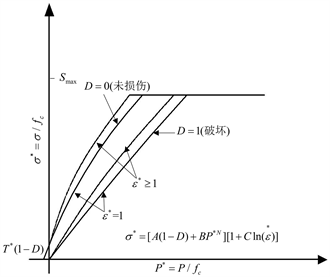Figure 1. The strength model of HJC modelFigure 2. The damage model of HJC model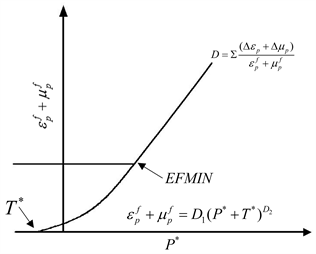Figure 3. The damage model of HJC model

3.2. RHT模型

1999年，Riedel  提出的模型是针对传统的劈裂压缩波传播规范而设计的，其中偏应力强度分别由弹性、失效和残余强度极限面组成，这一概念同时考虑了各向同性硬化、强度损失和刚度退化，这些极限面取决于压力、三轴应力状态和应变率，如图4所示。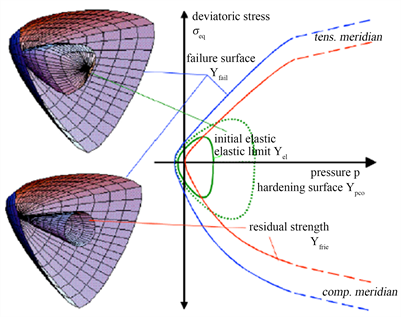(a)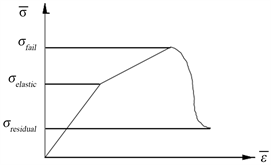(b)

Figure 4. RHT model. (a) Three limit surfaces and meridian. (b) Schematic diagram of “three stages”

RHT失效面方程为：

${\sigma }_{ep}^{*}\left(p,\theta ,\stackrel{˙}{\epsilon }\right)={Y}_{TXC}^{*}\left(p\right){R}_{3}\left(\theta \right){F}_{rate}\left(\stackrel{˙}{\epsilon }\right)$ (6)

${Y}_{TXC}^{*}\left(p\right)$ 为失效极限面上的等效强度函数， ${F}_{rate}\left(\stackrel{˙}{\epsilon }\right)$ 为应变率相关函数， ${R}_{3}\left(\theta \right)$ 为Lode角 $\theta$ 以及偏平面上失效曲线(失效面被偏平面相截后形成的曲线)在拉、压子午线处的偏应力之比 ${Q}_{2}$ 的函数，并且实验结果表明，混凝土的破坏强度与偏应力张量、第二不变量 ${J}_{2}$ 、第三不变量 ${J}_{3}$ 和静水压力有关，它们分别为

${Y}_{TXC}^{*}\left(p\right)=A{\left[{p}^{*}-{p}_{spall}^{*}{F}_{rate}\left(\stackrel{˙}{\epsilon }\right)\right]}^{N}$ (7)

${F}_{rate}\left(\stackrel{˙}{\epsilon }\right)=\left\{\begin{array}{l}{\left(\stackrel{˙}{\epsilon }/{\stackrel{˙}{\epsilon }}_{0}\right)}^{a}p\ge \frac{{f}_{c}}{3}\\ {\left(\stackrel{˙}{\epsilon }/{\stackrel{˙}{\epsilon }}_{0}\right)}^{\delta }p<\frac{{f}_{c}}{3}\end{array}$ (8)

${R}_{3}\left(\theta \right)=2\left(1-{Q}_{2}^{2}\right)\mathrm{cos}\theta +\left(2{Q}_{2}-1\right)\cdot {\left[4\left(1-{Q}_{2}^{2}\right){\mathrm{cos}}^{2}\theta +5{Q}_{2}^{2}-4{Q}_{2}\right]}^{1/2}/4\left(1-{Q}_{2}^{2}\right){\mathrm{cos}}^{2}\theta +{\left(1-2{Q}_{2}\right)}^{2}$ (9)

$\theta =\frac{1}{3}{\mathrm{cos}}^{-1}\left[\frac{3\sqrt{3}{J}_{3}}{2{J}_{2}^{3/2}}\right]\text{}0\le \theta \le \frac{\theta }{3}$

${Q}_{2}=\frac{{r}_{1}}{{r}_{c}}={Q}_{0}+{B}_{Q}{p}^{*}\text{}0.51\le {Q}_{2}\le 1.0$ (10)

3.3. Malvar (K&C)模型

Marlvar  等人采用Praudtl-Reuss流动法则采和8个独立参数定义3个固定极限面，以LLNL模型为基础，提出了K&C材料模型，该模型很好的描述了混凝土材料受压时的塑性流动。弹性极限面，失效极限面和残余强度极限面(如图5所示)，此外，该模型还将材料的硬化速率与损伤软化速率考虑其中，实验结果验证了该本构模型具有良好的吻合度。

$\Delta {\sigma }_{m}={a}_{0}+\frac{p}{{a}_{1}+{a}_{2}p}$ (11)

$\Delta {\sigma }_{r}=p/\left({a}_{1f}+{a}_{2f}p\right)$ (12)

$\Delta {\sigma }_{y}={\sigma }_{0y}+p/\left({a}_{1y}+{a}_{2y}p\right)$ (13)

$\Delta \sigma =\eta \left(\Delta {\sigma }_{m}-\Delta {\sigma }_{y}\right)+\Delta {\sigma }_{y}$ (14)

$\Delta \sigma =\eta \left(\Delta {\sigma }_{m}-\Delta {\sigma }_{r}\right)+\Delta {\sigma }_{r}$ (15)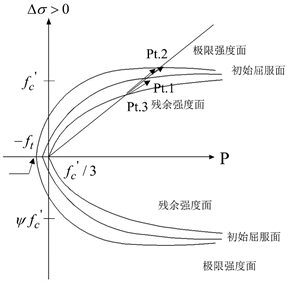Figure 5. The three failure surfaces of Malvar model

$\lambda =\left\{\begin{array}{l}\underset{0}{\overset{\stackrel{\to }{{\epsilon }_{p}}}{\int }}\frac{\text{d}\stackrel{\to }{{\epsilon }_{p}}}{{r}_{f}{\left(1+p/{r}_{f}{f}_{t}\right)}^{{b}_{1}}}\text{}p\ge 0\\ \underset{0}{\overset{\stackrel{\to }{{\epsilon }_{p}}}{\int }}\frac{\text{d}\stackrel{\to }{{\epsilon }_{p}}}{{r}_{f}{\left(1+p/{r}_{f}{f}_{t}\right)}^{{b}_{2}}}\text{}p<0\end{array}$ (16)

${f}_{d}=\left\{\begin{array}{l}1-\frac{|\sqrt{\frac{3{J}_{2}}{p}}|}{0.1}\text{}0\le \sqrt{\frac{3{J}_{2}}{p}<}0.1\\ 0\text{}\sqrt{\frac{3{J}_{2}}{p}}\ge 0.1\end{array}$ (17)

$\Delta \lambda ={b}_{3}{f}_{d}{k}_{d}\left({\epsilon }_{v}-{\epsilon }_{v,yield}\right)$ (18)

$\text{d}\stackrel{\to }{{\epsilon }_{p}}$ 表示塑性应变增量， ${r}_{f}$ 为单轴强度的应变率效应增强系数， ${b}_{1},{b}_{2}$ 分别为控制压缩和拉伸软化系数， ${f}_{t}$ 为拉伸强度， ${J}_{2}$ 是偏应力张量第二不变量， ${b}_{3}$ 控制体积拉伸软化系数， ${k}_{d}$ 是内部系数， ${\epsilon }_{v}$ 是拉伸体积应变， ${\epsilon }_{v,yield}$ 表示拉屈点体积应变。

3.4. ZWT模型

3.4.1. 经典的ZWT模型

ZWT  模型是由朱–王–唐在研究环氧树脂时提出的，该模型由一个非线性弹簧和两个描述不同特征时间的麦克斯韦体并联组成，如图6所示：

$\sigma ={\sigma }_{s}+{\sigma }_{m1}+{\sigma }_{m2}={E}_{0}\epsilon +\alpha {\epsilon }^{2}+\beta {\epsilon }^{3}+{E}_{1}{\int }_{0}^{t}\stackrel{˙}{\epsilon }\mathrm{exp}\left(-\frac{t-\tau }{{\phi }_{1}}\right)\text{d}\tau +{E}_{2}{\int }_{0}^{t}\stackrel{˙}{\epsilon }\mathrm{exp}\left(-\frac{t-\tau }{{\phi }_{2}}\right)\text{d}\tau$ (19)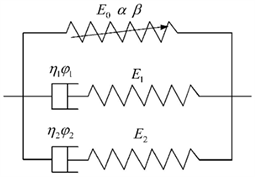Figure 6. The schematic diagram of ZWT modelTable 1. Maxwell related parameters

3.4.2. ZWT模型基础上建立的统计损伤本构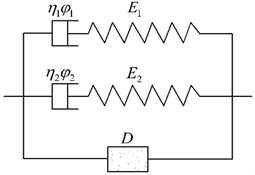Figure 7. Statistical damage constitutive model based on ZWT model

$\phi \left({\epsilon }_{a}\right)=\frac{m}{\alpha }{\epsilon }_{a}^{m-1}\mathrm{exp}\left(-\frac{{\epsilon }_{a}^{m}}{\alpha }\right)\left(\epsilon \ge 0\right)$ (20)

$D=1-\mathrm{exp}\left(-\frac{{\epsilon }_{a}^{m}}{\alpha }\right)\left(\epsilon \ge 0\right)$ (21)

${\sigma }_{a}={E}_{0}{\epsilon }_{a}\left(1-D\right)={E}_{0}{\epsilon }_{a}\mathrm{exp}\left(-\frac{{\epsilon }_{a}^{m}}{\alpha }\right)\left(\epsilon \ge 0\right)$ (22)

${\sigma }_{\text{1}}={E}_{1}{\int }_{0}^{t}\epsilon \mathrm{exp}\left(-\frac{t-\tau }{{\phi }_{1}}\right)\text{d}\tau$ (23)

${\sigma }_{\text{2}}={E}_{2}{\int }_{0}^{t}\epsilon \mathrm{exp}\left(-\frac{t-\tau }{{\phi }_{2}}\right)\text{d}\tau$ (24)

$\sigma ={\sigma }_{a}+{\sigma }_{1}+{\sigma }_{2}={E}_{0}{\epsilon }_{a}\mathrm{exp}\left(-\frac{{\epsilon }_{a}^{m}}{\alpha }\right)+{E}_{1}{\int }_{0}^{t}\epsilon \mathrm{exp}\left(-\frac{t-\tau }{{\phi }_{1}}\right)\text{d}\tau +{E}_{2}{\int }_{0}^{t}\epsilon \mathrm{exp}\left(-\frac{t-\tau }{{\phi }_{2}}\right)\text{d}\tau$ (25)

4. 准脆性材料动态本构关系的繁衍与发展

4.1. 弹塑性本构

1952年，Drucker和Prager对Von Mises准则进行了修正后，正式提出Drucker-Prager准则，分别引入自变量I1和静水压力J2用以描述材料的屈服特征和材料破坏特征，最终建立了经典的D-P  模型。周永强  在二次加载面理论的基础上，基于Drucker-Prager准则构建了二次加载面的应力路径模型。除此之外，将岩石材料受载过程中的刚度和强度的速率效应引入模型中，通过自编程序实现了岩石材料二次加载面模型的构建(模型的重要表达式详见表2)。Table 2. The important expressions of the elastoplastic constitutive model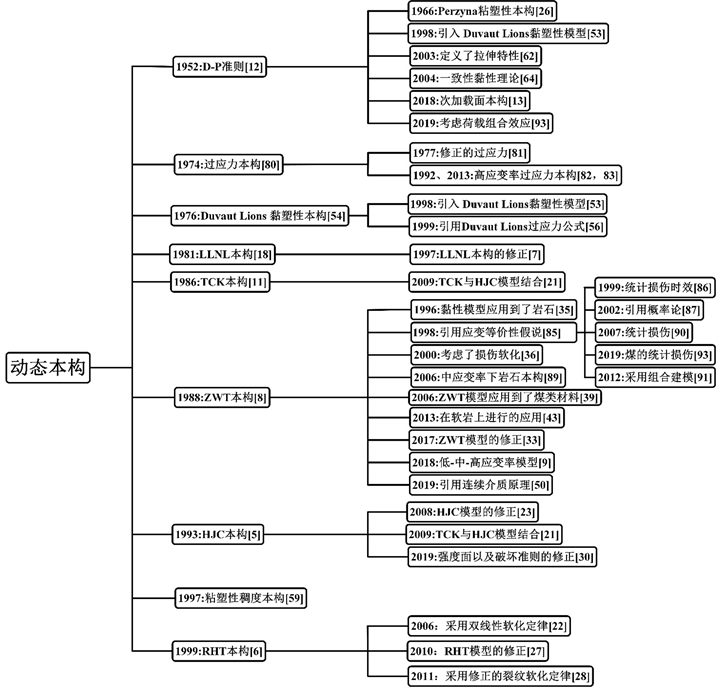Figure 8. Development of dynamic constitutive relation for quasi-brittle materials

4.2. 弹塑性损伤本构

Taylor  将Kipp-Grady模型与Budiansky  和Grady提出的等效体积模量、裂纹密度和碎块尺寸表达式结合，同时引入损伤因子，从而构建了提出经典的TCK模型。Suaris  等人基于动力平衡方程，提出了混凝土动态损伤本构模型。Hlomquist  提出了混凝土的计算本构模型(HJC)，该模型利用损伤、应变率和压力函数表示等效强度，体积应变率函数表示压力变化，塑性体积的函数表示损失类似过程。董毓利、谢和平  等人对混凝土材料进行了7个数量级范围内压缩试验，结果表明，随着应变率的逐渐增高，材料的峰值应力和峰值应变越来越大，但弹性模量并未发生明显的变化。此外，还建立了含有损伤因子w和内变量q的混凝土塑性损伤本构模型。Marlva模型是Marlvar  等人对LLNL模型  修正后提出的，在LLNL模型的基础上引入Praudtl-Reuss流动法则，并采用3个固定极限面和8个独立的参数定义材料的压缩子午线。Eibl1  在实验结果的基础上，证明了动态本构模型不仅仅需要考虑当前的应变率，同时需要考虑惯性的影响，通过以上定义，建立混凝土时效型弹塑性损伤本构。Burlion  用两个损伤标量描述弹性模量的退化：拉伸损伤变量和压缩损伤变量，使用修正的Gurson屈服函数和相关的流动准则，建立了塑性损伤本构模型。Liu  建立了新型塑性损伤本构模型，该模型是在TCK模型和HJC模型基础上构建的。Leppänen  借鉴RHT  模型，并在此基础上，采用了双线性软化定律，建立了修正的RHT塑性损伤本构模型。Polanco  基于HJC模型，首先在调整了应变率参数，其次引用了三个不同的损伤变量分别用于描述混凝土材料的拉伸、压缩和剪切机制，最后将第三偏应力不变量引入了本构模型中。在此基础上，建立了修正的HJC模型。刘海峰  基于混凝土冲击荷载作用下的实验研究，以修正Ottosen四参数破坏准则为屈服法则，引用Lemaitre应变等价性原理，同时考虑损伤，构建了混凝土动态塑性损伤模型。宁建国  在Perzyna  黏塑性本构方程的基础上，把统计细观理论与连续损伤理论结合在一起，建立动态塑性损伤本构模型。Zhen  在RHT模型的基础上，修正了残余强度面的Lode角依赖性、拉伸软化规律和动态拉伸强度函数，建立了修正的RHT动态塑性损伤本构模型。Nyström  使用修改后的裂纹软化定律和双线性主拉应力破坏准则，建立了修正的RHT塑性损伤本构模型。Wu  利用LS-DYNA有限元软件，修正了KCC模型的损伤变量，建立了修正的KCC塑性损伤本构模型。张社荣  对HJC模型的强度平面、应变率效应参数和破坏准则进行了修正，在此基础上建立了SHPB有限元模型(模型的重要表达式详见表3)。

Table 3. The important expressions of the elastoplastic damage constitutive model

4.3. 黏弹性本构Table 4. The important expressions of the viscoelastic constitutive model

4.4. 黏弹性损伤本构

Table 5. The important expressions of the viscoelastic damage constitutive model

4.5. 黏塑性本构

Perzyna模型是黏塑性模型的主要形式之一，Perzyna   基于参量变分原理以及Drucker屈服条件，建立了Perzyna黏塑性本构方程。Bicanic  在修改后的Perzyna弹/黏塑性理论的基础上，采用了应力率敏感的流动性参数和主应力空间中的两个表面(定义为偏离弹性的不连续表面和强度极限表面)作为损伤监测装置，以及假设应力点达到极限强度水平时就开始破坏不连续表面，建立了混凝土的速率和历史相关的黏塑性本构模型。López  采用DP破坏准则和Duvaut Lions  黏塑性模型，将应变率效应引入本构关系中，提出混凝土黏塑性本构方程。Kang H  研究了动态强度的增强和黏塑性的正则化特性，以便于解决拉伸中的应变软化问题。为此，将Kang和Willam  的弹塑性混凝土模型扩展到黏塑性Duvaut Lions过应力公式。Chen  考虑速率效应，保留初始边界值问题的适定性，同时引入应变软化，建立了高应变率下混凝土的黏塑性本构模型。Winnicki  在Wang  提出的黏塑性模型的基础上，建立了修正的黏性本构模型，该模型利用了各向同性形式的霍夫曼屈服函数，并进行了扩展，以包括拉伸和压缩过程中的单独的硬化/软化行为。Wang  将塑性应变率相关项引入屈服面方程，并通过塑性理论解释塑性变形，另外在本构模型中引入了率相关项，推导和构建了黏塑性本构模型。冯明珲  结合混凝土的变形特征，用简化后的统一本构模型模拟了混凝土非破坏截面的变形，取得了良好的效果。然后，应用统一的黏弹塑性本构模型对混凝土的跳跃试验进行了预测。陈书宇  研究动态荷载下混凝土的极限面的变化规律，以及塑性屈服面的变化特征，将Perzyma黏塑性和Bicanic塑性间断面运动理论用来描述混凝土的塑性特征，建立了混凝土黏塑性本构模型。Georgin  在Duvaut-Lions模型的基础上，定义了非光滑屈服准则(Rankine准则)，用于定义材料在拉伸特性，再通过Von-Mises准则或Drucker-Prager准则对双轴压缩建模，建立了黏塑性本构方程。肖诗云  从黏塑性模型的理论出发，对常用的DP模型进行了改进，在本构理论中引入了率效应，构建了基于DP准则的材料一致率型本构模型。肖诗云  根据一致性黏塑性模型理论，以及做了大量的混凝土动态压缩试验，在试验结果和Willam-Warke三参数本构模型的基础上，考虑应变率效应，推导出了修正的Willam-Warke三参数率相关本构模型。Pandey  提出了一种基于应变速率的混凝土黏塑性本构模型，用于分析瞬态动力载荷下的三维钢筋混凝土结构，并且该模型考虑了三维载荷情况下的压力敏感性和动态载荷情况下的应变率敏感性。褚卫江  在Winnicki和肖诗云工作的基础上，将线性 Drucker-Prager模型应用于广义的一致性黏塑性模型。Aráoz  建立的修正黏塑性本构模型源自于广义的Perzyna模型，该模型考虑体积和偏斜响应，是在小应变的连续力学框架内制定的现象学模型，并采用了盖函数和硬化函数(模型的重要表达式详见表6)。Table 6. The important expressions of the viscoplastic constitutive model

4.6. 黏塑性损伤本构Table 7. The important expressions of the viscoplastic damage constitutive model

4.7. 过应力模型

Lindholm  介绍了在Dresser玄武岩上进行的一系列测试的结果，并将获得的强度数据与包含温度和应变率的破坏准则相关联，建立了经典的过应力模型。20世纪80年代，川北  等人在过应力模型的基础上进行修正，得到修正的过应力模型，并且该模型可以很好的描述岩石的动态行为。于亚伦  采用先进的SHPB装置对四种矿岩进行了高应变率下的动载实验，并基于过应力理论与两个基本假设，提出了岩石过应力模型。谢理想  在修正过应力模型本构关系的基础上，通过量纲分析方法简化了修正的过应力本构关系，得到了修正的过应力本构模型(模型的重要表达式详见表8)。Table 8. The important expressions of the over stress model

4.8. 统计损伤模型

Li  通过考虑动能和表面能之间的平衡，以及加载速率，材料刚度和损伤的变化，基于连续介质力学和统计断裂力学，并假设岩石介质是各向同性，建立岩石统计损伤本构。曹文贵  基于应变等价性假说，考虑到岩石微观元素强度分布的随机性，建立了能够表示岩石微元强度分布的变量，构建了三维受载条件下岩石损伤软化本构方程和岩石损伤演化方程。单仁亮、东兆星  将统计损伤模型与黏弹性模型结合在一起，提出了统计损伤时效模型，假定损伤前损伤体均为线弹性的，并且强度均服从麦克斯韦分布。为了描述岩石的破碎分布以及损伤特性，徐未亚  将岩石损伤变量D引入本构模型中，并考虑弹塑性变形的特征，建立了岩石材料的统计损伤模型。单仁亮  通过同时将统计损伤本构模型和黏弹性模型的特点引入新的本构方程中，并对花岗岩和大理岩实验数据的分析，构建了一个简化的岩石统计损伤本构模型。李夕兵  利用组合模型的思路，将统计损伤模型的损伤特征和黏弹性模型的弹性特性归结在一起，建立了中等应变率下动载荷下一维和三维静载荷岩石的本构模型。杨明辉  经大量研究，得出了岩石微观元素强度的统计特征，考虑到岩石内部微观元素的复杂性，将这些微元体视为损伤体和的黏性结合体。同时考虑到动载荷作用下岩石的动态力学特性，建立了动荷载下岩石的统计损伤本构模型。刘军忠  引用前人研究方法，将统计损伤模型和黏弹性模型组合在一起，最终建立了基于麦克斯韦分布的动态损伤本构模型。曹文贵  在应变率影响的岩石动态强度准则的基础上，利用统计损伤理论和微元强度的测量方法，基于Lemaitre应变等价性假设，建立岩石动态荷载下的统计损伤本构模型。王恩元  引用了统计损伤理论，列举了三个基本假设来检验轴向静载荷，围压和冲击载荷对岩石动态力学性能影响，建立了岩石统计损伤本构模型(模型的重要表达式详见表9)。Table 9. The important expressions of the related statistical damage model

5. 结论与展望

NOTES

*通讯作者。

 王利民. 准脆性材料黏聚阻裂的计算与实验[J]. 力学季刊, 2013, 3(34): 456-462.

 王青原. 基于三点弯曲梁试验的准脆性材料断裂行为研究[D]: [硕士学位论文]. 贵阳: 贵州大学, 2018.

 王利民, 韩巍巍. 准脆性材料损伤破坏的细宏观联结分析[J]. 固体力学学报, 2015, 36(S1): 20-25.

 霍新. 基于Cosserat理论混凝土等准脆性材料弯曲性能尺寸效应[D]: [硕士学位论文]. 北京: 北京交通大学, 2018.

 Holmquist, T.J. and Johnson, G.R. (1993) A Computational Constitutive Model for Concrete Subjected to Large Strains, High Strain Rates and High Pressures. 14th International Symposium on Ballistics, Quebec, 26-29 September 1993, 591-600.

 Riedel, W. (1999) Penetration of Reinforced Concrete by BETA-B-500 Numerical Analysis Using a New Macroscopic Concrete Model for Hydrocodes. 9th International Symposium, Interaction of the Effects of Munitions with Structures, Berlin, 3-7 May 1999, 315-322.

 Malvar, L.J., Crawford, J.E., Wesevich, J.W., et al. (1997) A Plasticity Concrete Material Model for DYNA3D. International Journal of Impact Engineering, 19, 847-873.
https://doi.org/10.1016/S0734-743X(97)00023-7

 朱兆祥. 环氧树脂在高应变率下的热黏弹性本构方程和时温等效性[J]. 宁波大学学报, 1988, 1(1): 58-68.

 郭德勇, 吕鹏飞, 赵杰超. 煤岩冲击变形破坏特性及其本构模型[J]. 煤炭学报, 2018, 43(8): 2233-2242.

 Abrams, D.A. (1917) Effect of Rate of Application of Load on the Compressive Strength of Concrete. ASTM Journal, 17, 364-377.

 Taylor, L.M., Chen, E.P. and Kuszmaul, J.S. (1986) Microcrack-Induced Damage Accumulation in Brittle Rock under Dynamic Loading. Computer Methods in Applied Mechanics & Engineering, 55, 301-320.
https://doi.org/10.1016/0045-7825(86)90057-5

 许浒, 余志祥, 赵世春. 混凝土非线性分析中的非协调参数Drucker-Prager模型[J]. 四川大学学报(工程科学版), 2012, 44(4): 75-80.

 周永强, 盛谦, 罗红星. 考虑率效应的岩石材料次加载面动态本构模型[J]. 岩土工程学报, 2018, 40(10): 1818-1826.

 白冰, 李小春, 石露. 基于虚强度参数的塑性硬化模式[J]. 长江科学院院报, 2012, 29(8): 24-28.

 Budiansky, B. and O’Connell, R.J. (1976) Elastic Moduli of Cracked Solid. International Journal of Solids & Structures, 21, 61-72.
https://doi.org/10.1016/0020-7683(76)90044-5

 Suaris, W. (2016) Constitutive Model for Dynamic Loading of Concrete. Journal of Structural Engineering, 111, 563-576.
https://doi.org/10.1061/(ASCE)0733-9445(1985)111:3(563)

 董毓利. 不同应变率下混凝土受压全过程的实验研究及其本构模型[J]. 水利学报, 1997(7): 72-77.

 Livermore Software Technology Corporation (2003) LS-DYNA Keyword User’s Manual V970.

 Eibl, J. and Schmidt-Hurtienne, B. (1999) Strain-Rate-Sensitive Con-stitutive Law for Concrete. Journal of Engineering Mechanics, 125, 1411-1420.
https://doi.org/10.1061/(ASCE)0733-9399(1999)125:12(1411)

 Burlion, N., Gatuingt, F. and Pijaudier-Cabot, G. (2000) Compaction and Tensile Damage in Concrete: Constitutive Modelling and Application to Dynamic. Computer Methods in Applied Mechanics and Engineering, 183, 291-308.
https://doi.org/10.1016/S0045-7825(99)00223-6

 Liu, Y., Ma, A. and Huang, F. (2009) Numerical Simulations of Oblique-Angle Penetration by Deformable Projectiles into Concrete Targets. International Journal of Impact Engi-neering, 36, 438-446.
https://doi.org/10.1016/j.ijimpeng.2008.03.006

 Leppänen, J. (2006) Concrete Subjected to Projectile and Fragment Impacts: Modelling of Crack Softening and Strain Rate Dependency in Tension. International Journal of Impact Engineering, 32, 1828-1841.
https://doi.org/10.1016/j.ijimpeng.2005.06.005

 Polanco-Loria, M., Hopperstad, O.S., Børvik, T., et al. (2008) Numerical Predictions of Ballistic Limits for Concrete Slabs Using a Modified Version of the HJC Concrete Model. International Journal of Impact Engineering, 35, 290-303.
https://doi.org/10.1016/j.ijimpeng.2007.03.001

 刘海峰, 宁建国. 强冲击荷载作用下混凝土材料动态本构模型[J]. 固体力学学报, 2008, 29(3): 231-238.

 宁建国, 刘海峰, 商霖. 强冲击荷载作用下混凝土材料动态力学特性及本构模型[J]. 中国科学, 2008, 6(16): 759-772.

 Perzyna, P. (1966) Fundamental Problems in Viscoplasticity. Advances in Applied Mechanics, 9, 244-368.
https://doi.org/10.1016/S0065-2156(08)70009-7

 Tu, Z. and Lu, Y. (2010) Modifications of RHT Material Model for Improved Numerical Simulation of Dynamic Response of Concrete. International Journal of Impact Engi-neering, 37, 1072-1082.
https://doi.org/10.1016/j.ijimpeng.2010.04.004

 Nyström, U. and Gylltoft, K. (2011) Comparative Numerical Studies of Projectile Impacts on Plain and Steel-Fibre Reinforced Concrete. International Journal of Impact Engineering, 38, 95-105.
https://doi.org/10.1016/j.ijimpeng.2010.10.003

 Wu, J., Li, L., Du, X., et al. (2017) Numerical Study on the Asphalt Concrete Structure for Blast and Impact Load Using the Karagozian and Case Concrete Model. Applied Sciences, 7, 202-214.
https://doi.org/10.3390/app7020202

 张社荣, 宋冉, 王超. 碾压混凝土HJC动态本构模型修正及数值验证[J]. 振动与冲击, 2019, 38(12): 25-31.

 Izzuddin, B.A. and Fang, Q. (1997) Rate-Sensitive Analysis of Framed Structures Part I: Model Formulation and Verification. Structural Engineering & Mechanics, 5, 221-237.
https://doi.org/10.12989/sem.1997.5.3.221

 黄海健. 轻质泡沫混凝土动态力学性能及本构关系[J]. 建筑材料学报, 2020, 23(2): 232-238.

 Zhang, H., Wang, B., Xie, A., et al. (2017) Experimental Study on Dynamic Mechanical Properties and Constitutive Model of Basalt Fiber Reinforced Concrete. Construction and Building Materials, 152, 154-167.
https://doi.org/10.1016/j.conbuildmat.2017.06.177

 尚仁杰. 混凝土动态本构行为研究[D]: [博士学位论文]. 大连: 大连理工大学, 1994.

 郑永来, 夏颂佑. 岩石黏弹性连续损伤本构模型[J]. 岩石力学与工程学报, 1996(S1): 428-432.

 陈江瑛. 水泥砂浆的率型本构方程[J]. 宁波大学学报, 2000, 27(2): 1-5.

 胡时胜, 王道荣. 冲击载荷下混凝土材料的动态本构关系[J]. 爆炸与冲击, 2002, 22(3): 242-246.

 商霖, 宁建国. 强冲击载荷下混凝土动态本构关系[J]. 工程力学, 2005, 22(2): 116-119.

 单仁亮. 云驾岭煤矿无烟煤的动态本构模型研究[J]. 岩石力学与工程学报, 2006, 25(11): 2258-2263.

 宁建国, 商霖, 孙远翔. 混凝土材料冲击特性的研究[J]. 力学学报, 2006, 38(2): 199-208.

 孟益平. 冲击载荷作用下混凝土的率型本构关系[J]. 安徽理工大学学报, 2007, 27(4): 15-18.

 翟越, 赵均海, 李寻昌. 岩石类材料损伤黏弹塑性动态本构模型研究[J]. 岩石力学与工程学报, 2011, 30(S2): 3820-3824.

 谢理想. 软岩及混凝土材料损伤型黏弹性动态本构模型研究[J]. 岩石力学与工程学报, 2013, 32(1): 857-864.

 Zhang, H., Liu, Y., Sun, H., et al. (2016) Transient Dynamic Be-havior of Polypropylene Fiber Reinforced Mortar under Compressive Impact Loading. Construction and Building Ma-terials, 111, 30-42.
https://doi.org/10.1016/j.conbuildmat.2016.02.049

 Zhang, H., Wang, L., Zheng, K., et al. (2018) Research on Compressive Impact Dynamic Behavior and Constitutive Model of Polypropylene Fiber Reinforced Concrete. Con-struction and Building Materials, 187, 584-595.
https://doi.org/10.1016/j.conbuildmat.2018.07.164

 张文清, 穆朝民. 突出煤的冲击力学行为及本构关系的研究[J]. 煤矿安全, 2016, 47(7): 1-4.

 Dong, S., et al. (2018) Dynamic Impact Behaviors and Constitutive Model of Super-Fine Stainless Wire Reinforced Reactive Powder Concrete. Construction and Building Materials, 184, 602-616.
https://doi.org/10.1016/j.conbuildmat.2018.07.027

 焦楚杰, 李习波, 程从密. 基于分形理论的高强混凝土动态损伤本构关系[J]. 爆炸与冲击, 2018, 38(4): 925-930.

 付玉凯, 解北京, 王启飞. 煤的动态力学本构模型[J]. 煤炭学报, 2013, 38(10): 1769-1774.

 解北京, 严正. 基于层叠模型组合煤岩体动态力学本构模型[J]. 煤炭学报, 2019, 44(2): 463-472.

 Perzyna, P. (1963) The Constitutive Equations for Rate Sensitive Plastic Materials. Quarterly of Applied Mathematics, 20, 321-332.
https://doi.org/10.1090/qam/144536

 Biani, N. and Zienkiewicz, O.C. (1983) Constitutive Model for Concrete under Dynamic Loading. Earthquake Engineering & Structural Dynamics, 11, 689-710.
https://doi.org/10.1002/eqe.4290110508

 López Cela, J.J. (1998) Analysis of Reinforced Concrete Structures Subjected to Dynamic Loads with a Viscoplastic Drucker-Prager Model. Applied Mathematical Modelling, 22, 495-515.
https://doi.org/10.1016/S0307-904X(98)10050-1

 Duvaut, G.L.J.L. (1976) Inequalities in Mechanics and Physics. Springer, Berlin.
https://doi.org/10.1007/978-3-642-66165-5

 Kang, H.D. and Willam, K.J. (2000) Performance Evaluation of Elastoviscoplastic Concrete Model. Journal of Engineering Mechanics, 126, 995-1000.
https://doi.org/10.1061/(ASCE)0733-9399(2000)126:9(995)

 Kang, H.D. and Willam, K.J. (1999) Localization Characteristics of Triaxial Concrete Model. Journal of Engineering Mechanics, 125, 941-950.
https://doi.org/10.1061/(ASCE)0733-9399(1999)125:8(941)

 Chen, D., Al-Hassani, S.T.S., Yin, Z., et al. (2001) Modeling Shock Loading Behavior of Concrete. International Journal of Solids & Structures, 38, 8787-8803.
https://doi.org/10.1016/S0020-7683(01)00102-0

 Winnicki, A., Pearce, C.J. and Bieanie, N. (2001) Visco-plastic Hoffman Consistency Model for Concrete. Computers & Structures, 79, 7-19.
https://doi.org/10.1016/S0045-7949(00)00110-3

 Wang, W.M. (1997) Stationary and Propagative Instabilities in Metals. Delft University of Technology, Delft.

 冯明珲, 吕和祥, 林皋. 黏弹塑性理论在混凝土变形中的应用[J]. 工程力学, 2002, 19(2): 3-8.

 陈书宇. 动态载荷下的混凝土本构关系及有限元实现[J]. 辽宁工学院学报, 2003, 23(1): 5-7.

 Georgin, J.F. and Reynouard, J.M. (2003) Modeling of Structures Subjected to Impact: Concrete Behaviour under High Strain Rate. Cement and Concrete Composites, 25, 131-143.
https://doi.org/10.1016/S0958-9465(01)00060-9

 肖诗云, 林皋, 王哲. Drucker-Prager材料一致率型本构模型[J]. 工程力学, 2003, 20(4): 147-151.

 肖诗云, 林皋, 李宏男. 混凝土WW三参数率相关动态本构模型[J]. 计算力学学报, 2004, 21(6): 641-646.

 Pandey, A.K., Kumar, R., Paul, D.K., et al. (2006) Strain Rate Model for Dynamic Analysis of Reinforced Concrete Structures. Journal of Structural Engineering, 132, 1393-1401.
https://doi.org/10.1061/(ASCE)0733-9445(2006)132:9(1393)

 褚卫江, 苏静波, 徐卫亚. 基于一致性理论的Drucker-Prager材料弹黏塑本构模型[J]. 岩土力学, 2008, 29(3): 811-816.

 Aráoz, G. and Luccioni, B. (2015) Modeling Concrete like Materials under Sever Dynamic Pressures. International Journal of Impact Engineering, 76, 139-154.
https://doi.org/10.1016/j.ijimpeng.2014.09.009

 李兆霞. 一个综合模糊裂纹和损伤的混凝土应变软化本构模型[J]. 固体力学学报, 1995, 16(1): 22-30.

 Govindjee (1995) Anisotropic Modelling and Numerical Simulation of Brittle Damage in Concrete. International Journal for Numerical Methods in Engineering, 38, 3611-3633.
https://doi.org/10.1002/nme.1620382105

 Ju, J.W. (1997) Discussion: Rate Dependent Damage Model for Concrete in Dynamics. Journal of Engineering Mechanics, 123, 1326-1328.
https://doi.org/10.1061/(ASCE)0733-9399(1997)123:12(1326)

 Rossi, P. (1997) Strain Rate Effects in Con-crete Structures: The LCPC Experience. Materials and Structures, 30, 54-62.
https://doi.org/10.1007/BF02539277

 陈书宇. 一种混凝土损伤模型和数值方法[J]. 爆炸与冲击, 1998, 18(4): 62-70.

 Lee, J. and Fenves, G.L. (1998) A Plastic-Damage Concrete Model for Earthquake Analysis of Dams. Earthquake Engineering & Structural Dynamics, 27, 937-956.
https://doi.org/10.1002/(SICI)1096-9845(199809)27:9<937::AID-EQE764>3.0.CO;2-5

 陆晓霞, 张培源. 在围压冲击条件下岩石损伤黏塑性本构关系[J]. 重庆大学学报(自然科学版), 2002, 25(1): 6-8.

 Gatuingt, F. and Pijaudier, C.G. (2002) Coupled Damage and Plasticity Modelling in Transient Dynamic Analysis of Concrete. In-ternational Journal for Numerical and Analytical Methods in Geomechanics, 26, 1-24.
https://doi.org/10.1002/nag.188

 Ragueneau, F. and Gatuingt, F. (2003) Inelastic Behavior Modelling of Concrete in Low and High Strain Rate Dynamics. Computers & Structures, 81, 1287-1299.
https://doi.org/10.1016/S0045-7949(03)00043-9

 Ren, X. and Li, J. (2013) A Unified Dynamic Model for Concrete Considering Viscoplasticity and Rate-Dependent Damage. International Journal of Damage Mechanics, 22, 530-555.
https://doi.org/10.1177/1056789512455968

 Marzec, I. and Tejchman, J. (2013) Computational Modelling of Concrete Behaviour under Static and Dynamic Conditions. Bulletin of the Polish Academy of Sciences: Technical Sciences, 61, 85-96.
https://doi.org/10.2478/bpasts-2013-0007

 Ju, J.W. (1997) Discussion: Rate Dependent Damage Model for Concrete in Dynamics. Journal of Engineering Mechanics, 123, 1326-1328.
https://doi.org/10.1061/(ASCE)0733-9399(1997)123:12(1326)

 Lindholm, U.S., Yeakley, L.M. and Nagy, A. (1974) The Dynamic Strength and Fracture Properties of Dresser Basalt. International Journal of Rock Mechanics & Mining Sciences & Geomechanics Abstracts, 11, 181-191.
https://doi.org/10.1016/0148-9062(74)90885-7

 Muxia, Z., Zuoteng, Y. and Chuanbei, R. (1977) On the Me-chanical Behaviour of Rocks under Impulsive Loading. Bulletin of the Faculty of Engineering, Hokkaido University, 3, 51-61.

 于亚伦. 高应变率下的岩石本构方程[C]//岩石破碎理论与实践——全国第五届岩石破碎学术会论文选集. 岩石破碎理论与实践,1992: 40-44.

 谢理想, 赵光明, 孟祥瑞. 岩石在冲击载荷下的过应力本构模型研究[J]. 岩石力学与工程学报, 2013, 32(S1): 2772-2781.

 Liu, L. and Katsabanis, P.D. (1997) Development of a Continuum Damage Model for Blasting Analysis. International Journal of Rock Mechanics & Mining Sciences, 34, 217-231.
https://doi.org/10.1016/S0148-9062(96)00041-1

 曹文贵. 岩石损伤软化统计本构模型之研究[J]. 岩石力学与工程学报, 1998, 17(6): 628-633.

 东兆星, 单仁亮. 高应变率下岩石本构特性的研究[J]. 工程爆破, 1999, 5(2): 5-9.

 徐卫亚, 韦立德. 岩石损伤统计本构模型的研究[J]. 岩石力学与工程学报, 2002, 21(6): 787-791.

 单仁亮. 岩石动态破坏的时效损伤本构模型[J]. 岩石力学与工程学报, 2003, 22(11): 1771-1776.

 李夕兵, 左宇军, 马春德. 中应变率下动静组合加载岩石的本构模型[J]. 岩石力学与工程学报, 2006, 25(5): 865-874.

 杨明辉, 赵明华, 曹文贵. 基于统计理论的岩石动态损伤本构模型研究[J]. 武汉理工大学学报, 2007, 29(4): 95-98.

 刘军忠, 许金余, 吕晓聪. 围压下岩石的冲击力学行为及动态统计损伤本构模型研究[J]. 工程力学, 2012, 29(1): 55-63.

 曹文贵, 林星涛, 张超. 基于非线性动态强度准则的岩石动态变形过程统计损伤模拟方法[J]. 岩石力学与工程学报, 2017, 36(4): 794-802.

 王恩元, 孔祥国, 何学秋. 冲击载荷下三轴煤体动力学分析及损伤本构方程[J]. 煤炭学报, 2019, 44(7): 2049-2056.

Top